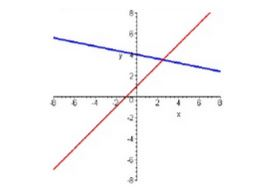Ontario Tech acknowledges the lands and people of the Mississaugas of Scugog Island First Nation.

We are thankful to be welcome on these lands in friendship. The lands we are situated on are covered by the Williams Treaties and are the traditional territory of the Mississaugas, a branch of the greater Anishinaabeg Nation, including Algonquin, Ojibway, Odawa and Pottawatomi. These lands remain home to many Indigenous nations and peoples.

We acknowledge this land out of respect for the Indigenous nations who have cared for Turtle Island, also called North America, from before the arrival of settler peoples until this day. Most importantly, we acknowledge that the history of these lands has been tainted by poor treatment and a lack of friendship with the First Nations who call them home.

This history is something we are all affected by because we are all treaty people in Canada. We all have a shared history to reflect on, and each of us is affected by this history in different ways. Our past defines our present, but if we move forward as friends and allies, then it does not have to define our future.

# Solving Linear Systems

Sometimes, we are interested in solving two linear equations simultaneously for x and y...graphically, this means finding the point of intersection of two lines. There are two methods commonly used in high school when solving a system of equations, so let's review these. The first method is solving by substitution and the second is solving by elimination...either one can be used, and they will of course give you the same results.## Solving by Substitution

Let's start by taking a closer look at solving a system of equations using the substitution method. The method is as follows:

• Solve one of the equations for one of the variables (i.e., isolate the variable);
• Substitute the equation from step 1 into the other equation;
• You should now have an equation in one variable, so solve it; finally,
• Substitute the solution from step 3 into one of the equations to solve for the other variable.

Example: Solve for x and y using the method of substitution.

1) -x + y = 4

2) x + 2y = 5

Solution:

Rearrange equation 1 to solve for y:

y = 4 + x

Substitute the rearranged equation into equation 2:

x + 2(4 + x) = 5

x + 8 + 2x = 5

3x = -3

x = -1

Sub x into equation 1:

-(-1) + y = 4

1 + y = 4

y = 3

Therefore, x = -1 and y =3

Note: In the example above, equation 1 was rearranged to solve for y. However, the same final answer would have been reached regardless of which equation was rearranged and which variable was isolated. In the last step, the solution for x was substituted into equation 1 to solve for y; however, it could have been substituted into equation 2 just as easily.

## Solving by Elimination

Now we will take a look at solving a system of equation using the elimination method. The elimination method involves removing one of the variables from the equations through the use of either addition or subtraction. The method can be summarized as follows:

• Multiply all terms of one or both equations by appropriate factors so that both equations will have the same coefficient for one of the variables;
• Add or subtract the two new equations to eliminate one of the variables;
• Solve for the resulting variable; and
• Substitute the solution from step 3 into one of the original equations to solve for the other variable.

Example: Solve for x and y using the method of elimination.

1) -x + y =4

2) x + 2y = 5

Solution:

Add equation 1 to equation 2:

-x + y + x + 2y = 4+5

3y = 9

y = 3

Sub y into equation 1:

-x + (3) = 4

-x = 1

x = -1

Therefore, x = -1 and y = 3.

Note: In the above example, neither of the equations needed to be manipulated in order to have a term eliminated from the equations, since -x + x = 0. Therefore, the two equations were simply added together. In the last step, note that we could also have substituted y = 3 into equation 2 to solve for x.

Example 1 (Substitution):

Example 2 (Elimination):

Example 3 (Elimination):

Setting Up and Solving Linear Systems (Application):

Solving a Linear System business word problem (Application):